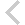FICO Xpress Optimization Examples Repository
 FICO Optimization Community FICO Xpress Optimization HomeFolio - Modelling examples from 'Getting started'

Description
• Chapter 3 Inputting and Solving a Linear Programming problem
• foliolp.mos: modeling and solving a small LP problem
• foliolperr.mos: LP model with syntax errors
• foliolps.mos: LP model using string indices
• Chapter 4 Working with data
• foliodata.mos (data file: folio.dat): data input from file, result output to a file, model parameters
• folioodbc.mos (data files: folio.xls, folio.mdb, folio.sqlite): data input from a spreadsheet or database, result output to a spreadsheet or database, model parameters
• folioexcel.mos (data file: folio.xls): same as folioodbc.mos but with Excel-specific data input and output (Windows only)
• foliosheet.mos (data file: folio.xls): same as folioodbc.mos but with data input and output through generic spreadsheet access
• foliocsv.mos (data file: folio.csv): same as folioodbc.mos but with data input and output through generic spreadsheet access in CSV format
• Chapter 5 Drawing user graphs
• folioloop.mos (data files: folio.dat, foliodev.dat): re-solving with varied parameter settings
• folioloop_graph.mos (data files: folio.dat, foliodev.dat): re-solving with varied parameter settings, graphical solution display
• foliolps_graph.mos: same as foliolps, adding graphical solution display
• Chapter 6 Mixed Integer Programming
• foliomip1.mos (data file: folio.dat): modeling and solving a small MIP problem (binary variables)
• foliomip2.mos (data file: folio.dat): modeling and solving a small MIP problem (semi-continuous variables)
• folioqp.mos (data file: folioqp.dat): modeling and solving a QP and a MIQP problem
• folioqp_graph.mos (data files: folioqp.dat, folioqpgraph.dat): re-solving a QP problem with varied parameter settings, graphical solution display
• folioqc.mos (data file: folioqp.dat): modeling and solving a QCQP and
• foliomiqc.mos (data file: folioqp.dat): modeling and solving a MIQCQP
• Chapter 8 Heuristics
• folioheur.mos (data file: folio.dat): heuristic solution of a MIP problem

Source Files

Data Files

folioqpgraphr.mos

```(!******************************************************
Mosel R Example Problems
========================

file folioqpgraphr.mos
``````````````````````
Modeling a small QP problem
to perform portfolio optimization.
Minimize variance subject to different target return.
-- Using R to calculate covariance matrix --
-- Graphical output via R --

!!! This example requires an installation of R, see the
!!! chapter 'R' of the 'Mosel Language Reference' for
!!! compatible versions and setup instructions

(c) 2015 Fair Isaac Corporation
author: S.Lannez, Jul. 2015, rev. Sep. 2017
*******************************************************!)

model "Portfolio optimization with QP, graphical output"
uses "mmxprs", "mmnl"
uses "mmsystem"
uses "r"                            ! Use R functions

parameters
MAXVAL = 0.3     ! Max. investment per share
MINAM = 0.5      ! Min. investment into N.-American values
GRDEVICE = "png" ! Set to 'png' or 'pdf' to change the type of document.
end-parameters

declarations
SHARES = 1..10                     ! Set of shares
RISK: set of integer               ! Set of high-risk values among shares
NA: set of integer                 ! Set of shares issued in N.-America
DATES: set of string               ! Historical dates
RET: array(SHARES) of real         ! Estimated return in investment
VAR: array(SHARES,SHARES) of real  ! Variance/covariance matrix of
! estimated returns
OPEN: array(SHARES,DATES) of real  ! Historical share value at market opening
CLOSE: array(SHARES,DATES) of real ! Historical share value at market closing
SOLRET: array(range) of real       ! Solution values (total return)
SOLDEV: array(range) of real       ! Solution values (average deviation)
end-declarations

initializations from "folioqp.dat"
RISK RET NA
end-initializations

! Load historical values to compute the covariance
initializations from "folioqphist.dat"
OPEN CLOSE
end-initializations

! **** Perfom some statistics using R ****

! Copy array to R environments
Rset('open',OPEN)
Rset('close',CLOSE)
! Print covariance of share value at market openings
writeln("Covariances at market openings:")
Rprint('cov(t(open))')
! Calculate and retrieve covariance of mean value
Rgetarr('cov(t((open+close)/2))',VAR)

! **** Mathematical model ****

declarations
frac: array(SHARES) of mpvar       ! Fraction of capital used per share
end-declarations

! Objective: mean variance
Variance:= sum(s,t in SHARES) VAR(s,t)*frac(s)*frac(t)

! Minimum amount of North-American values
sum(s in NA) frac(s) >= MINAM

! Spend all the capital
sum(s in SHARES) frac(s) = 1

! Upper bounds on the investment per share
forall(s in SHARES) frac(s) <= MAXVAL

! Solve the problem for a range of returns: this is the efficient frontier
target:= min(s in SHARES) RET(s)
RMAX:= max(s in SHARES) RET(s)

while(target < RMAX) do
Return:= sum(s in SHARES) RET(s)*frac(s) >= target    ! Target yield
minimize(Variance)                ! Solve the problem

if (getprobstat = XPRS_OPT) then  ! Save the optimal solution value
ct+=1
SOLDEV(ct):= getobjval
SOLRET(ct):= target
writeln("Solution for target ", target, "%")
else
writeln("No solution for target return >= ", target, "%")
break
end-if
target += 1
end-do

! **** Drawing graphs to represent results and data ****

declarations
DEV: array(SHARES) of real         ! Standard deviation
NAMES: array(SHARES) of string     ! Names of shares
t: text
end-declarations

initializations from "folioqpgraph.dat"
NAMES ! DEV
end-initializations

! Compute the standard deviation
forall(s in SHARES) DEV(s) := sqrt(VAR(s,s))

! Setting up the R environment
Reval('require(graphics)')          ! plot() and heatmap() functions
Reval('require(grDevices)')         ! heatmap dependency

if (GRDEVICE.size>0) then
Reval(GRDEVICE+'("folioqpgraphr%03d.'+GRDEVICE+'")') ! Open graphical file
end-if

! **** Drawing the efficient frontier ****

! Copy arrays to R
Rset('solval',SOLRET)
Rset('soldev',SOLDEV)

! Plot the graphs
Reval('plot(solval,soldev,color="black",type="l",'+
'main="Portfolio Return",'+
'xlab="Solution Value",ylab="Solution Variance")')
Reval('points(solval,soldev,pch=21,cex=1,col="dark red")')
if (GRDEVICE.size=0) then           ! Wait for user entry
Reval('dev.flush()')               ! Print plots
writeln("Press enter to continue...")
end-if

! Clean up arrays
Reval('rm(solval)')
Reval('rm(soldev)')

! **** Drawing the shares characteristics ****

! Copy arrays to R
Rset('ret',RET)
Rset('dev',DEV)
Rset('names',NAMES)
Rset('risk',RISK)
Rset('shares',SHARES)

! Create the color vectors
Reval('h <- c()')
Reval('h[shares] <- "green"')
Reval('h[risk] <- "red"')

! Plot the graphs
Reval('plot(ret,dev,col=h,bg=h,type="p",pch=21,'+
'main="Shares",'+
'xlab="Share Value",ylab="Share Deviation",'+
'xlim=c(-5,max(ret)+5),ylim=c(min(dev)-5,max(dev)+5))')
Reval('legend("topleft",c("high risk","low risk"),'+
'col=c("red","green"),pch=21)')
Reval('text(ret,dev,names,pos=3)')
if (GRDEVICE.size=0) then           ! Wait for user entry
Reval('dev.flush()')               ! Print plots
writeln("Press enter to continue...")
end-if

! Clean up arrays
forall(ar in {"ret","dev","risk","shares"})
Reval('rm('+ar+')')

! **** Drawing the correlation heat map ****

Reval('z <- cor(t(open+close)/2)')  ! Compute the correlation matrix
Reval('rownames(z) <- names')       ! Set the name of the rows
Reval('colnames(z) <- names')       ! Set the name of the columns
Rprint('symnum(z)')                 ! Textual representation of the correlation
Reval('heatmap(z,Rowv=NA,Colv=NA,symm=TRUE,main="Share Value Correlation")')
if (GRDEVICE.size=0) then           ! Wait for user entry
Reval('dev.flush()')               ! Print plots
writeln("Press enter to continue...")